# Making Excel Charts, Formulas, and Tables with Python—PyderPuffGirls Episode 8

In this last post of the series, I will continue on automating Excel with Python and show you how to use a few commands outside the Home Tab.

In this post, I will show you how to

• Insert image
• Make charts
• Write formula
• Make tables

and conclude the series with a productivity tip in data science.

## Installation

Like the previous post, I will use `openpyxl` to make the Excel spreadsheet. But there is one more library to install if you want to follow this guide: PIL (Python Image Library). Otherwise, you will see this error: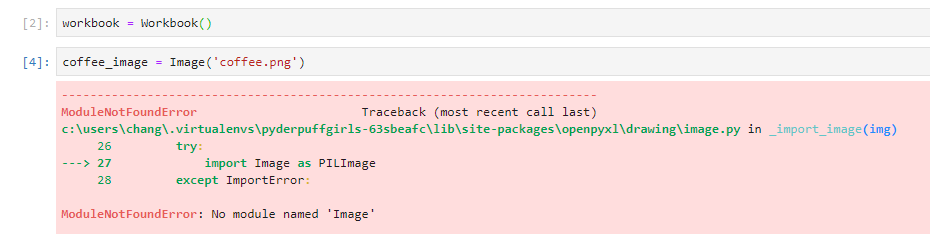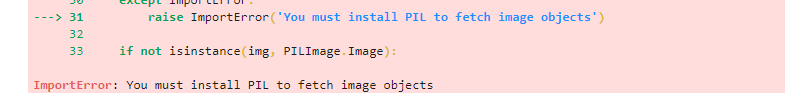When I googled it, latest version of PIL was for Python 1.5.2—wtf? So, do not pip install PIL. What I need to install was the `Pillow` library:

``````pip install --user Pillow
``````

## Inserting image

To insert an image from disk, I can make an `Image` object, then add the image to worksheet by anchoring the image on its top left cell.

For example, in this piece of code, I put an image on cell `C2`:

``````from openpyxl import Workbook
from openpyxl.drawing.image import Image

workbook = Workbook()

coffee_image = Image('coffee.png')

worksheet['C1'] = 'Me drinking coffee and kicking back'

workbook.save('example.xlsx')
workbook.close()
``````

and the result looks like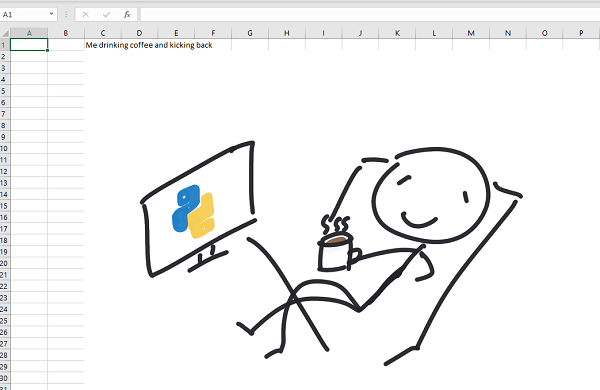### Scaling image

I can also modify the `Image` before I insert to scale my image. In particular, I want to make changes to two attributes:

• height
• width

For example, I can the height to 50% of the original image in my code:

``````coffee_image = Image('coffee.png')
coffee_image.height = coffee_image.height * 0.5
``````

As a result, the spreadsheet looks like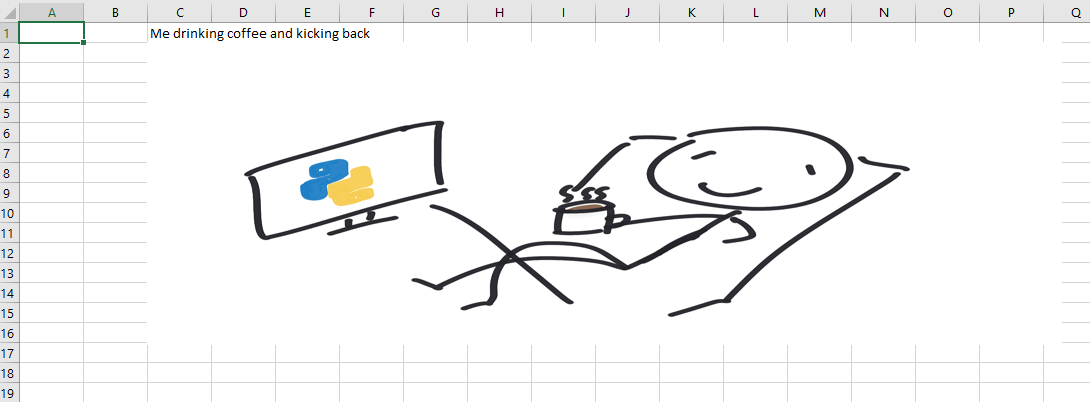## Charts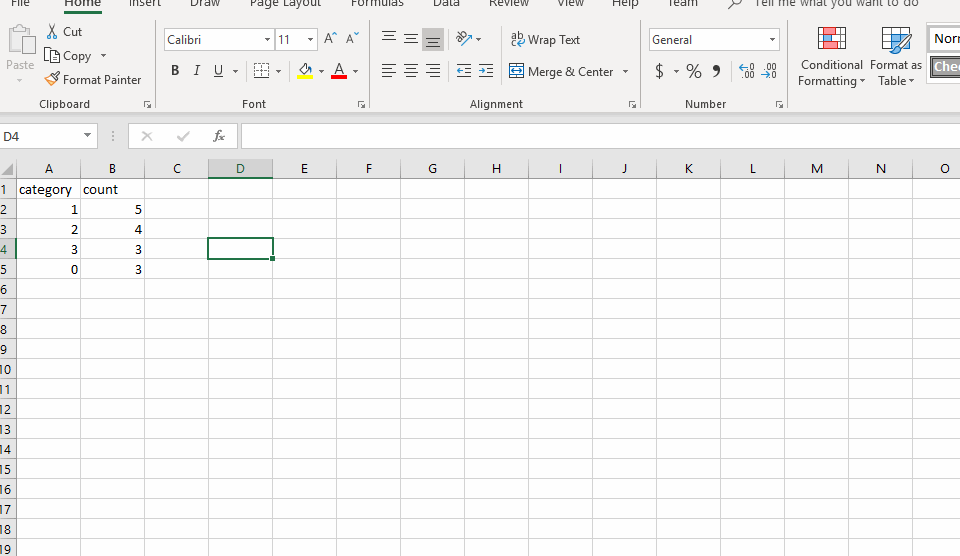`openpyxl` only supports a limited number of charts. To see the full list of supported charts, please refer to the documentation

### Making charts

Charts in Excel are a way to display referenced data. So my goal here is to set a range of cells (say, a rectangular grid of cells) that I want to plot with.

Let’s say I have some data called `cp_count` (count of first 15 rows of the UCI heart disease data.)

``````from openpyxl.utils.dataframe import dataframe_to_rows
from openpyxl.chart import BarChart, Series, Reference

cp_count = pd.DataFrame({
'category': {0: 1, 1: 2, 2: 3, 3: 0},
'count': {0: 5, 1: 4, 2: 3, 3: 3}
})

workbook2 = Workbook()
worksheet = workbook2.active

for r in dataframe_to_rows(cp_count, index=False, header=True):
worksheet.append(r)
``````

Once I wrote the data to the worksheet, I can make a chart at cell `D3` by setting the reference of my bar chart to the corresponding data cells:

``````sheet = worksheet.title

chart = BarChart()

# range_string use the format
# sheetname!top-left:bottom-right to select data
data = Reference(worksheet, range_string=f'{sheet}!B1:B5')
category = Reference(worksheet, range_string=f'{sheet}!A2:A5')

chart.set_categories(category)

workbook2.save('chart_example.xlsx')
``````

to get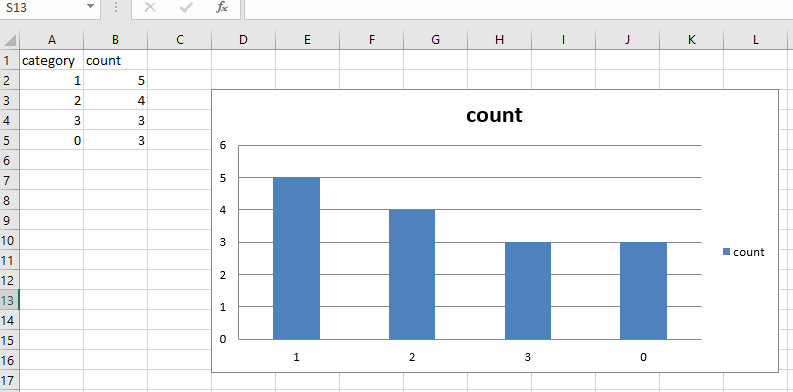I used `range_string` here to select a rectangular box for my data. There is another way to select the range of data by defining a box with the column and row numbers:

``````data = Reference(ws, min_col=2, min_row=1, max_row=5, max_col=2)
category = Reference(ws, min_col=1, min_row=2, max_row=5)
``````

Omitting one of the max or min means we are selecting on only one column or a row. The resulted chart is the same.

### Chart attributes

Based on the documentation, there seems to be a few attributes I can tune for my charts

#### Style

``````chart.style = 10
``````

then it creates a slightly different chart with white boundaries around the bars. I have yet found any documentation on which number maps to what style in Excel—so if you figured it out, let me know.

#### Unknown attributes

There are a few attributes in openpyxl’s chart page that I found wasn’t doing anything visible. i.e.

``````chart.type = 'col'
chart.shape = 10
``````

## Writing Formula

I can write an Excel formula by setting a cell to the correct formula name and data range. For example, to calculate the sum of column B, I can call the `SUM` function in Excel by writing

``````worksheet['B6'] = '=SUM(B2:B5)'
``````

which gives me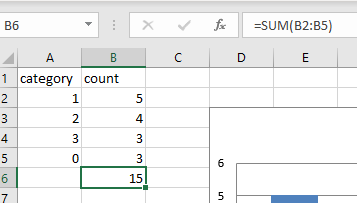## Format as table

A table in Excel, like charts, is a reference to a group of cells. In `openpyxl`, there is a `Table` object that I can style by the same table style name from Excel.

For example, if I want to use Table Style Light 1 from Excel on the same data that I used for `BarChart`, I can first find the name from Excel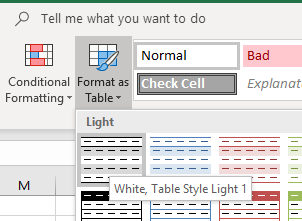then I can write

``````# data, workbook, and worksheet are the same as in the BarChart example
tab = Table(displayName="Table1", ref="A1:B5")

# I list out the 4 show-xyz options here for reference
style = TableStyleInfo(
name="TableStyleLight1",
showFirstColumn=False,
showLastColumn=False,
showRowStripes=True,
showColumnStripes=False
)
tab.tableStyleInfo = style

workbook2.save('table_example.xlsx')
``````

and it gives me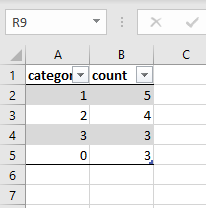For documentation, see

## The End

Thank you for reading through all 8 posts of PyderPuffGirls! (And thanks Keith, for coming up with a name for our sessions!)

This series of posts came from my observation that many people in analytics tried Python but did not see how it can help them in their job. I hope that through these posts, you have found at 2-3 things that you can use to automate away the boring parts of an analytics job—that can be reinvested to more challenge and rewarding work.

Therefore, I want to close this post with a final trick:

### The final trick

Even if you have made yourself more efficient through automation:

because is tempting to give the users what they want once it is ready.

If I can get a report in 5 minutes, then I can email them the result on minute 6, right? But this is a terrible pattern—new tasks and requests arrive the moment you send the old one out.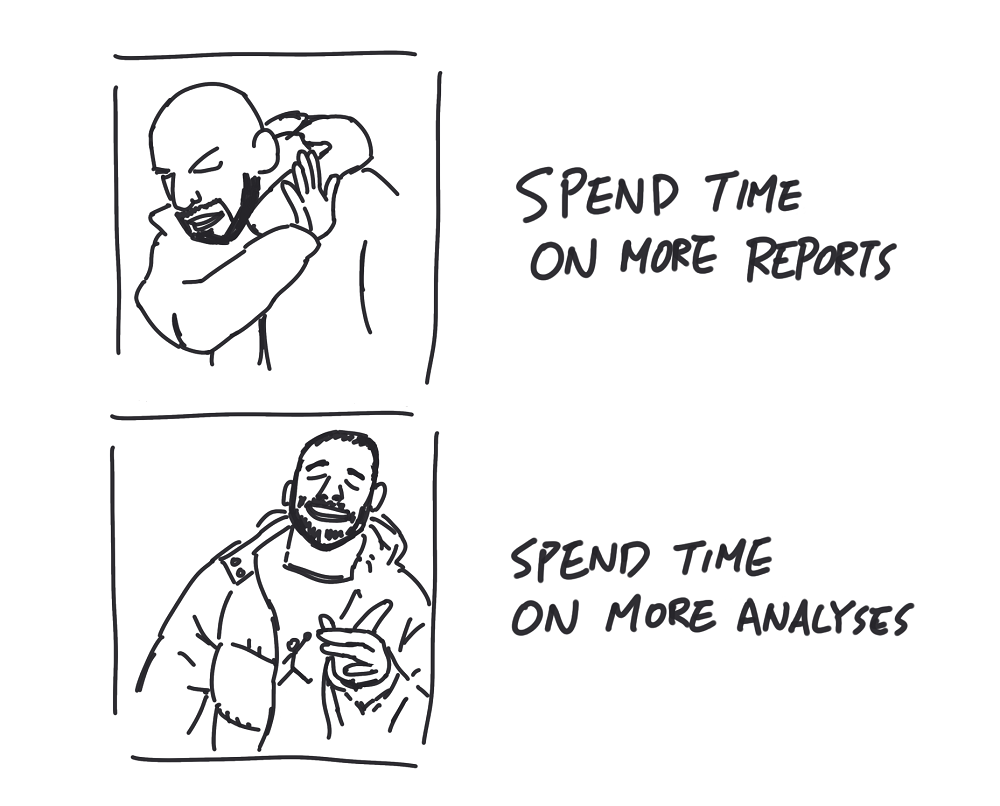Instead of giving the users what they want, give them what they need. Send the reports out on the same schedule to buy you extra time. Use the extra time on problems that only the human mind can solve to discover and develop data insights. I think this is where the real fun in data science is. So let’s put an end to the boring stuff and have fun.

More PyderPuffGirls